# Selina Solutions Concise Mathematics Class 6 Chapter 15: Decimal Fractions Exercise 15(C)

Selina Solutions Concise Mathematics Class 6 Chapter 15 Decimal Fractions Exercise 15(C) help students with the fundamental concepts of multiplication and division of decimal numbers. Students can clear their doubts instantly, by referring to the solutions PDF. The Solutions are prepared by subject experts, keeping in mind the important questions that would appear in the exams. Students can boost their logical thinking in solving complex problems with the help of solutions PDF. Selina Solutions Concise Mathematics Class 6 Chapter 15 Decimal Fractions Exercise 15(C), PDF links which are provided here

## Selina Solutions Concise Mathematics Class 6 Chapter 15: Decimal Fractions Exercise 15(C) Download PDF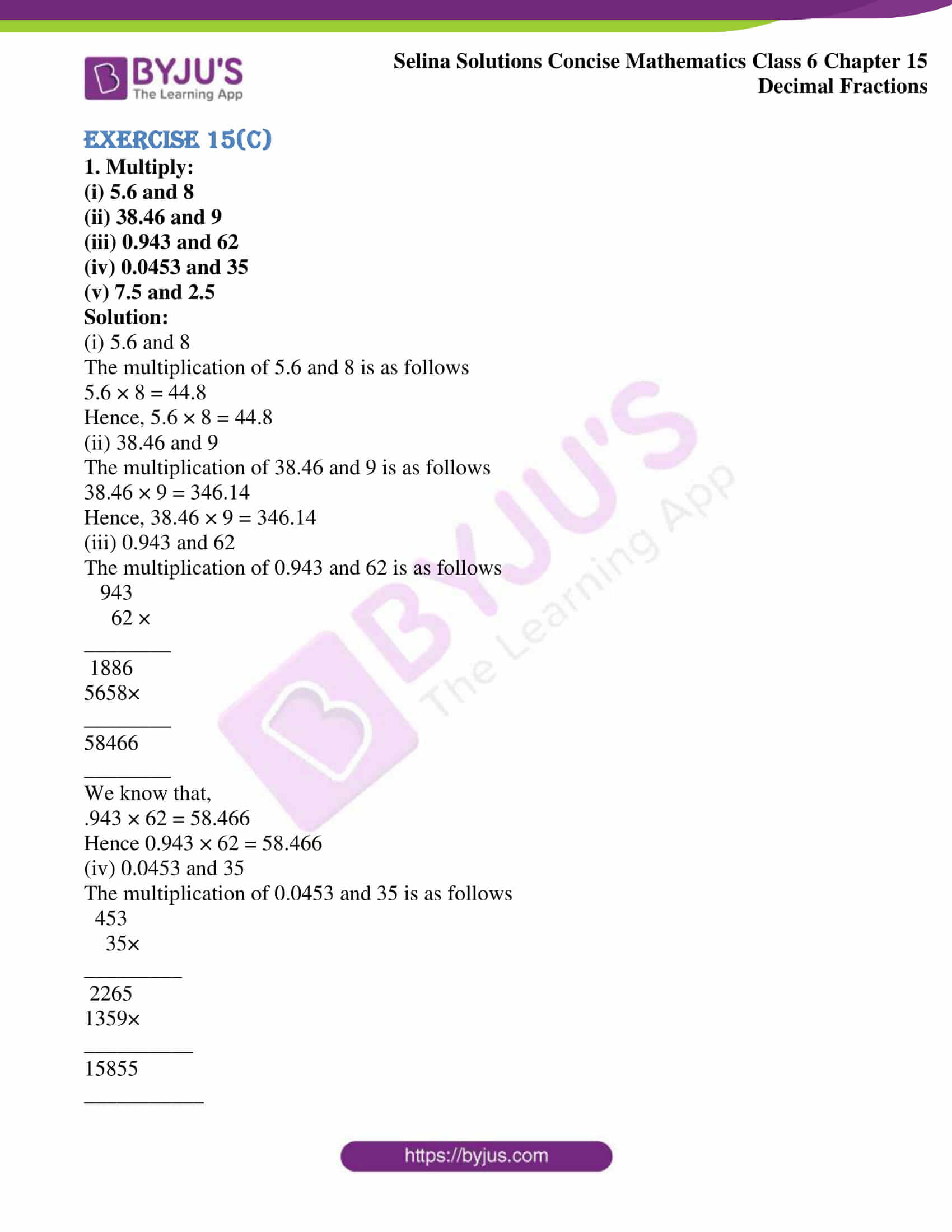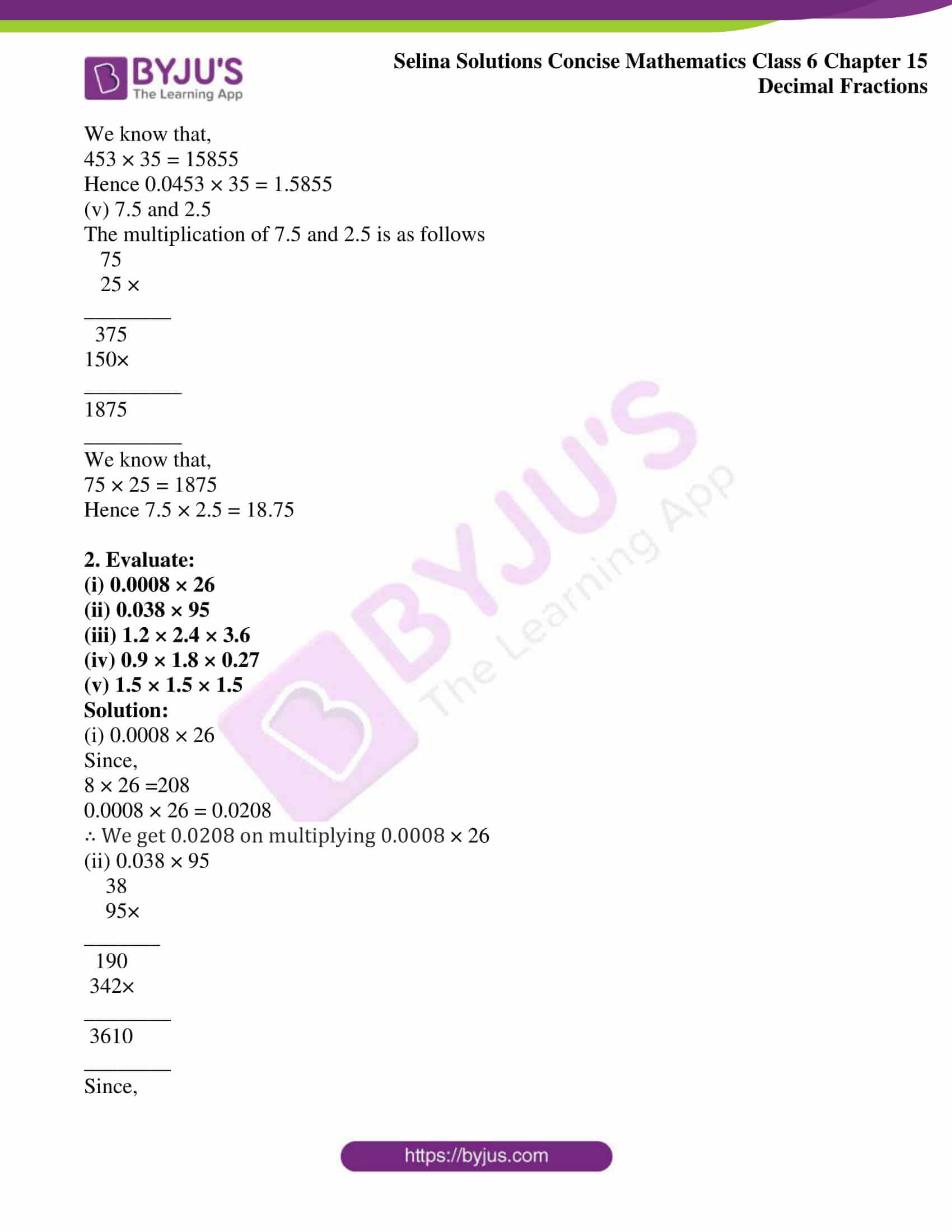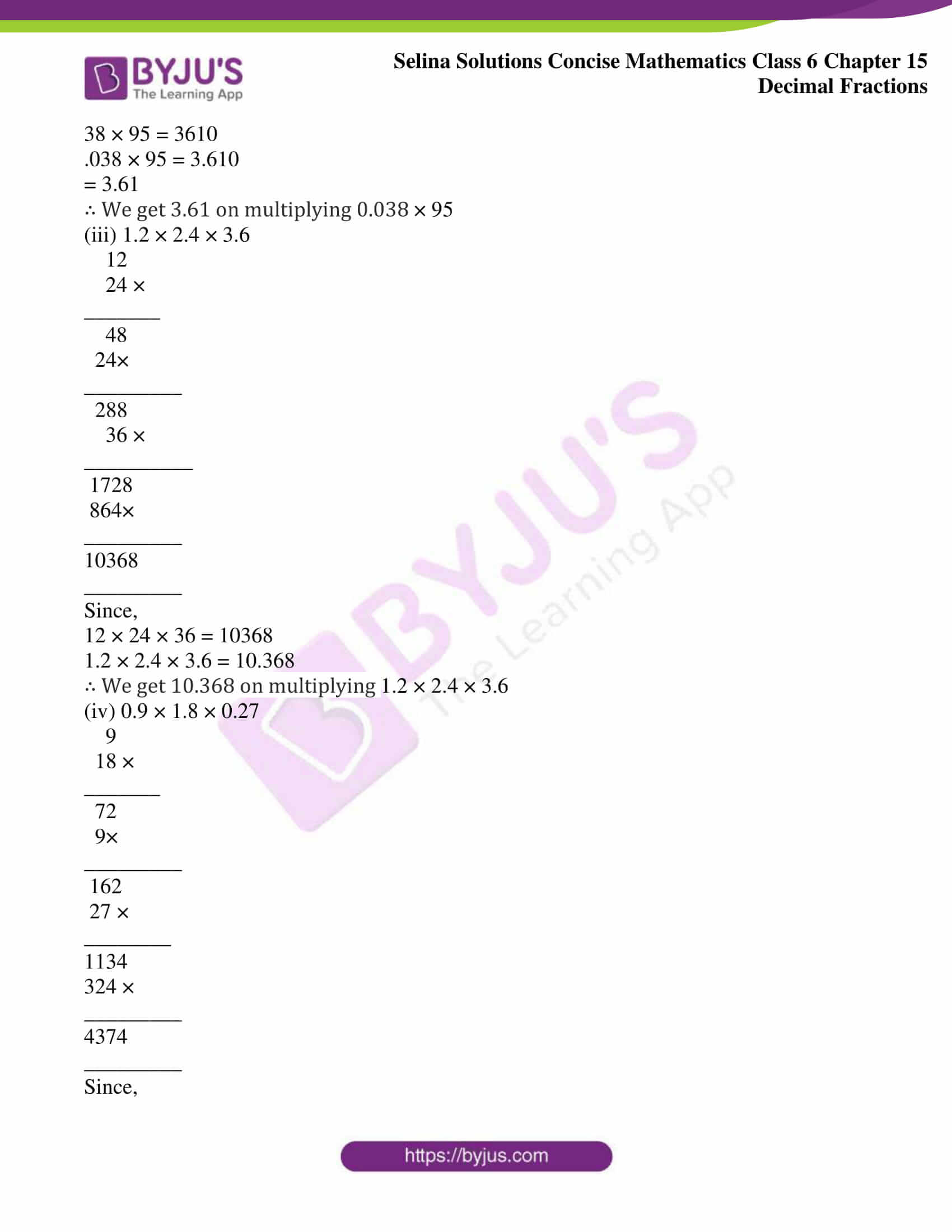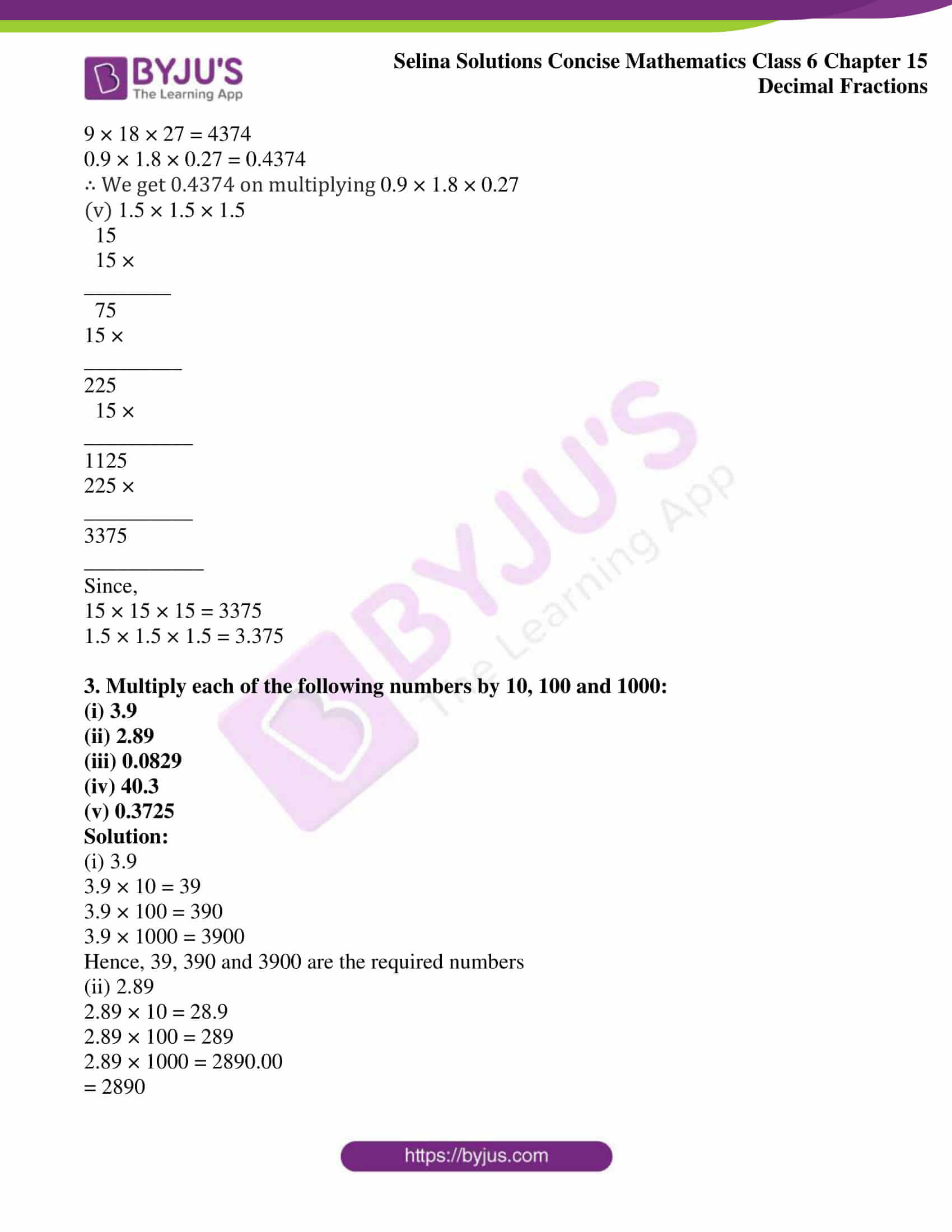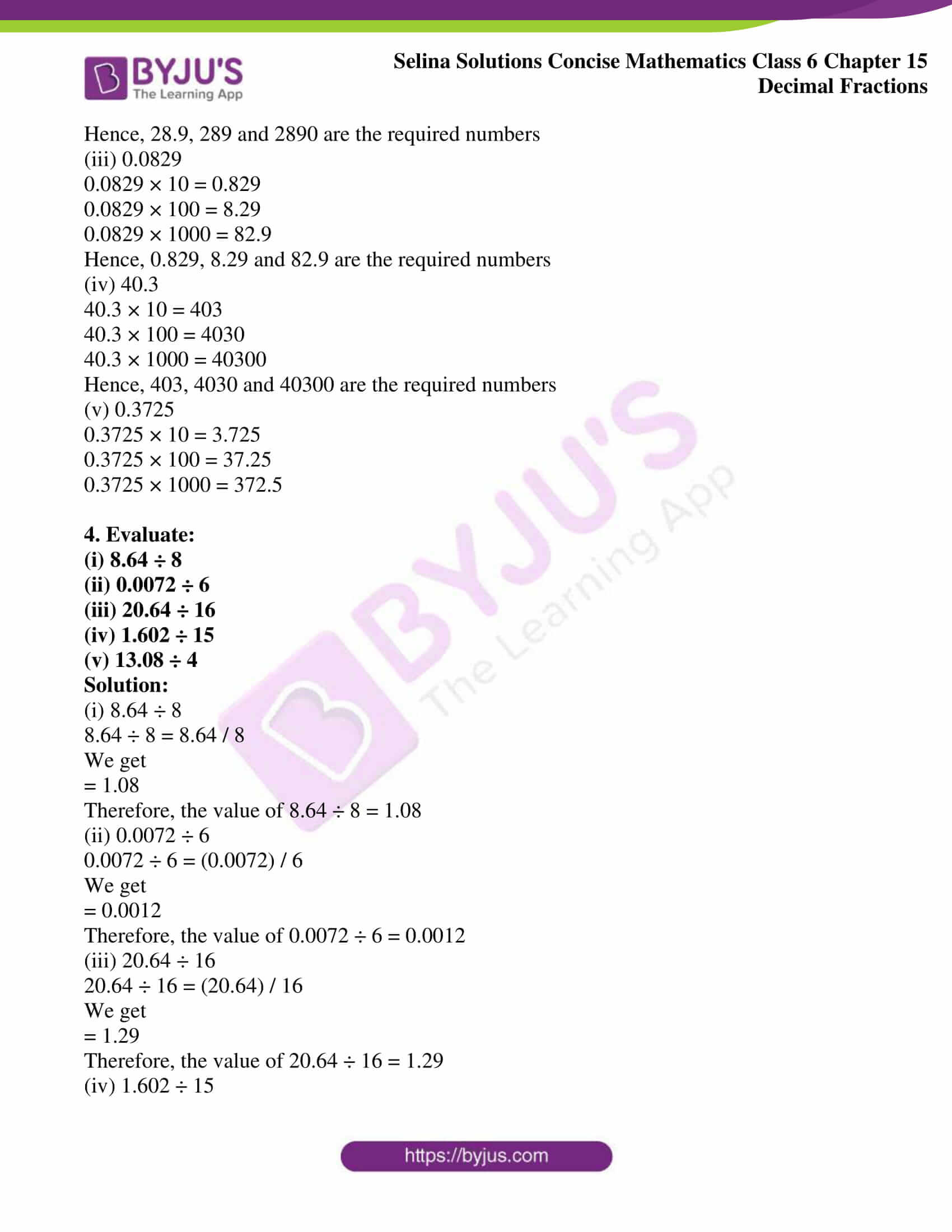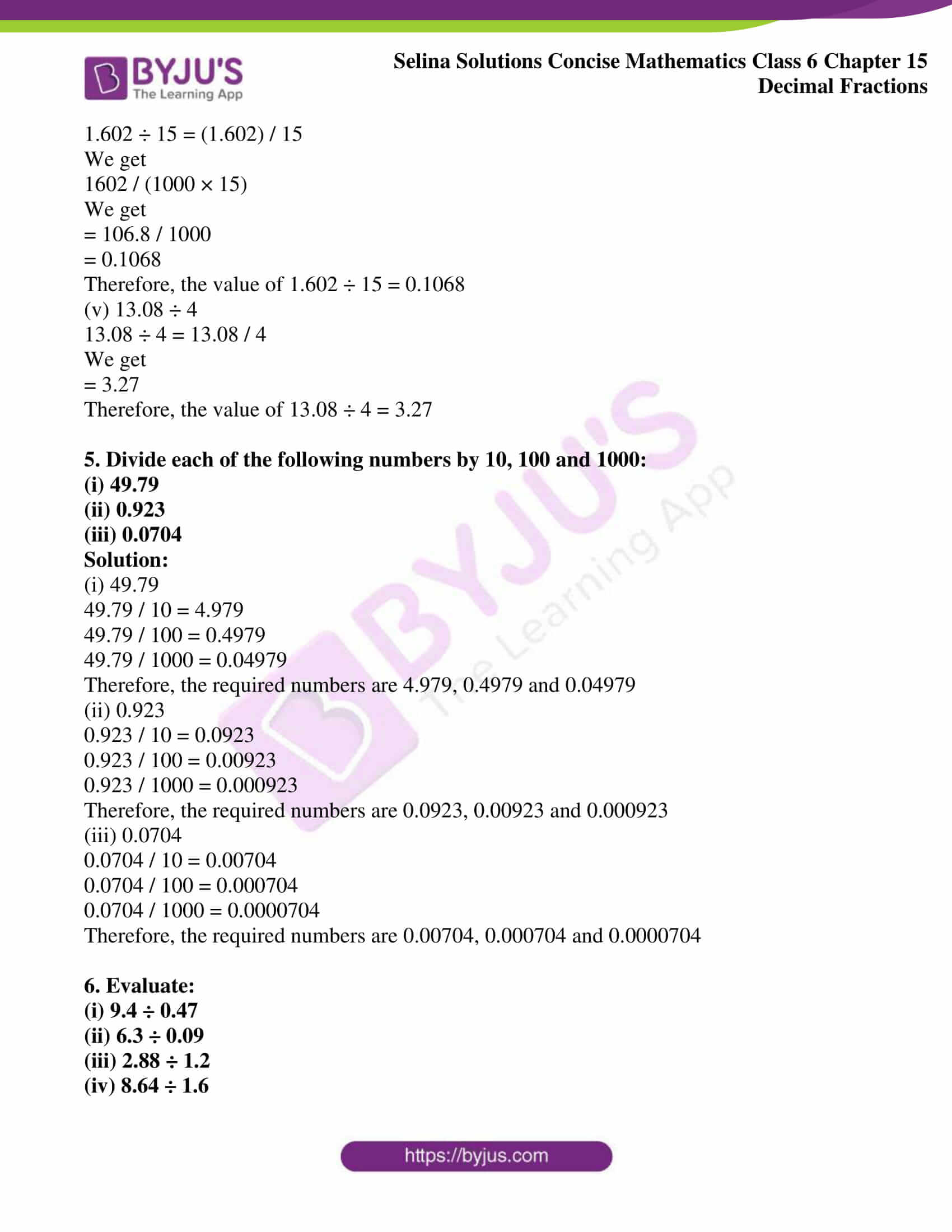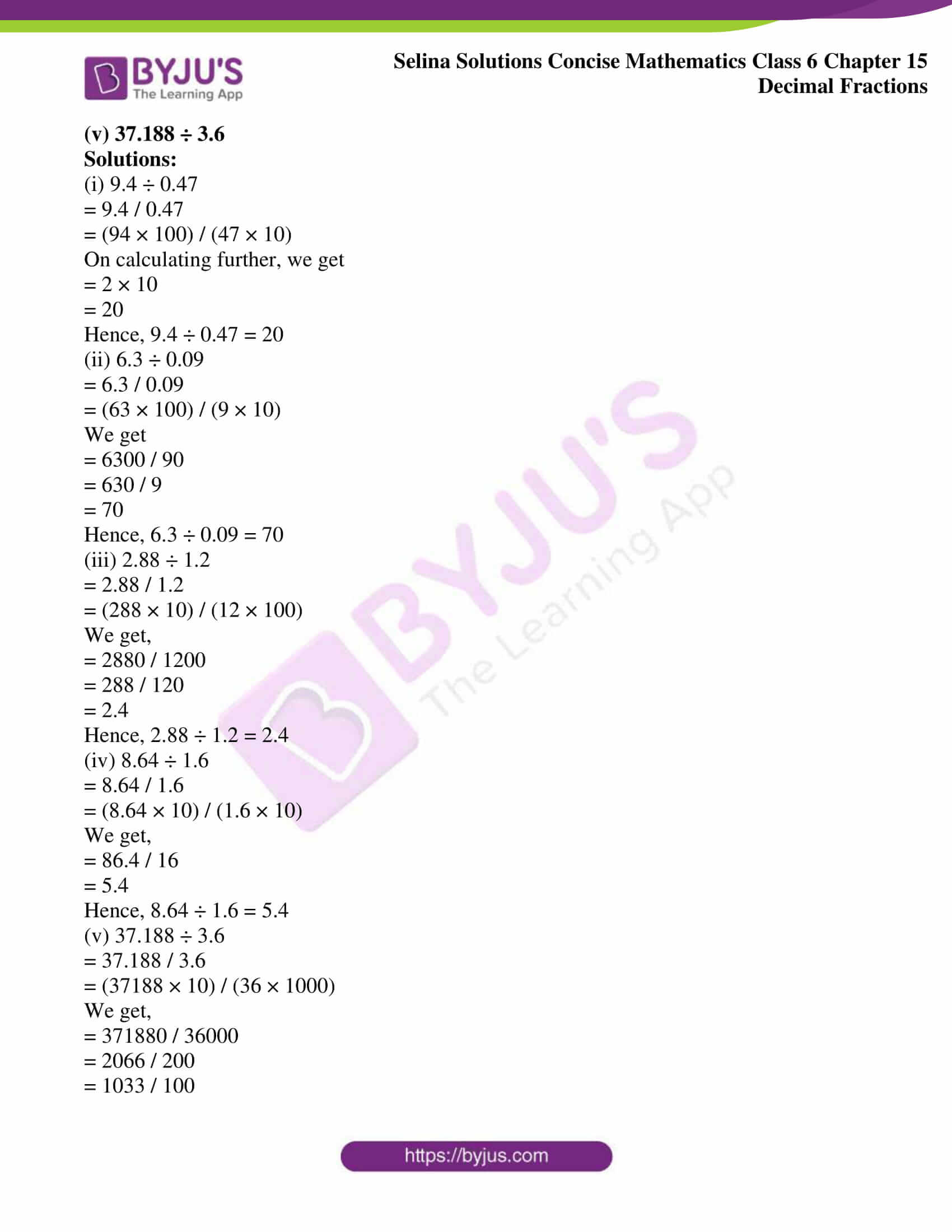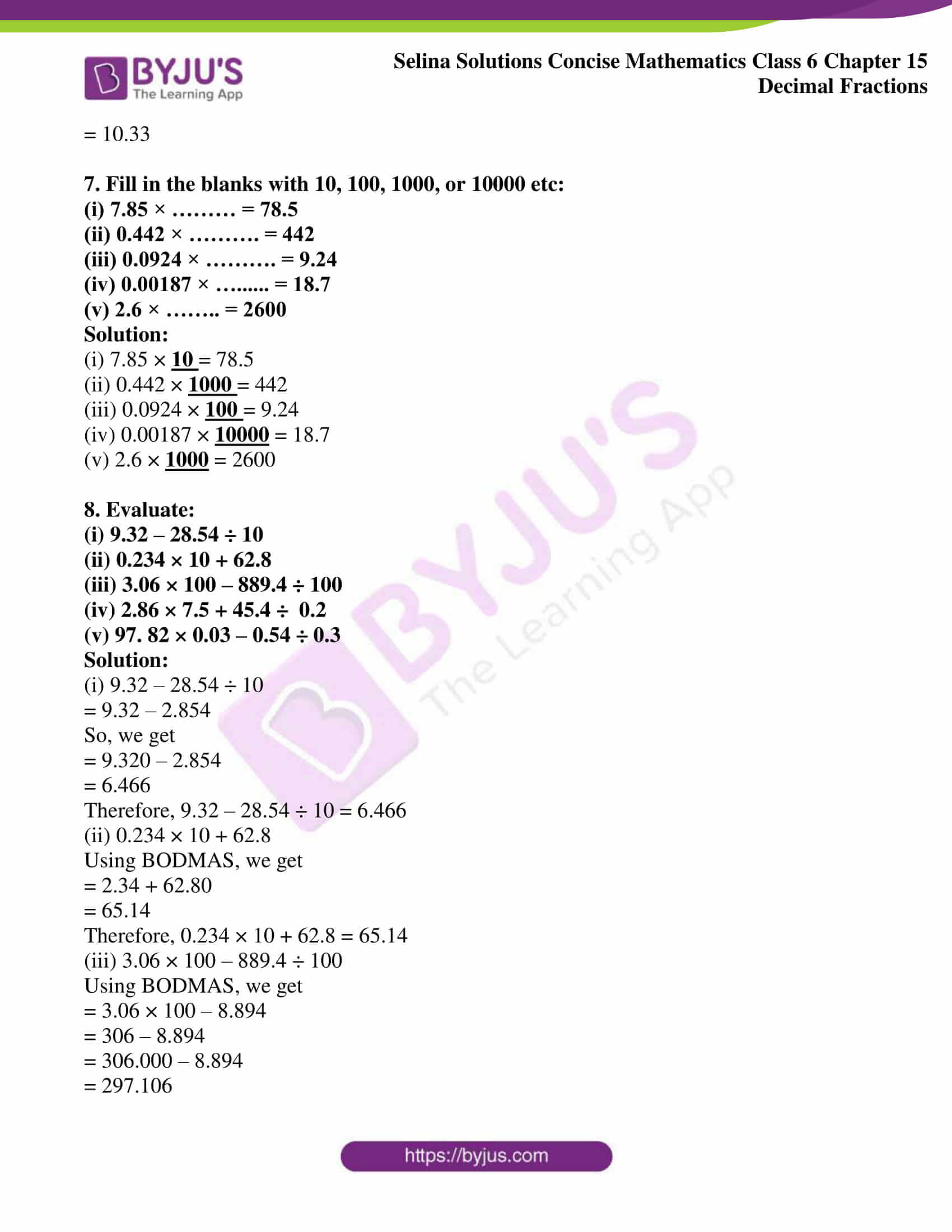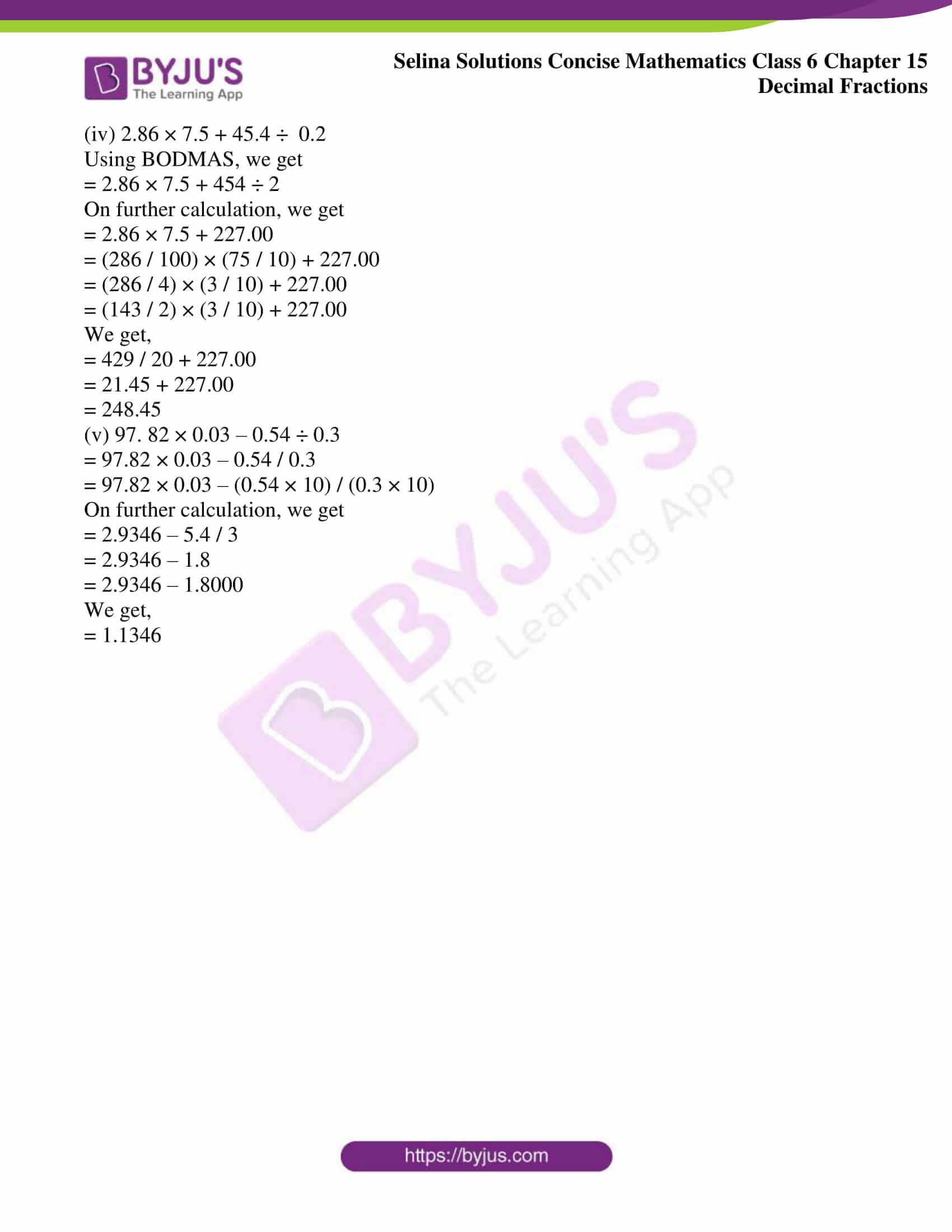### Access Selina Solutions Concise Mathematics Class 6 Chapter 15: Decimal Fractions Exercise 15(C)

Exercise 15(C)

1. Multiply:

(i) 5.6 and 8

(ii) 38.46 and 9

(iii) 0.943 and 62

(iv) 0.0453 and 35

(v) 7.5 and 2.5

Solution:

(i) 5.6 and 8

The multiplication of 5.6 and 8 is as follows

5.6 × 8 = 44.8

Hence, 5.6 × 8 = 44.8

(ii) 38.46 and 9

The multiplication of 38.46 and 9 is as follows

38.46 × 9 = 346.14

Hence, 38.46 × 9 = 346.14

(iii) 0.943 and 62

The multiplication of 0.943 and 62 is as follows

943

62 ×

________

1886

5658×

________

58466

________

We know that,

.943 × 62 = 58.466

Hence 0.943 × 62 = 58.466

(iv) 0.0453 and 35

The multiplication of 0.0453 and 35 is as follows

453

35×

_________

2265

1359×

__________

15855

___________

We know that,

453 × 35 = 15855

Hence 0.0453 × 35 = 1.5855

(v) 7.5 and 2.5

The multiplication of 7.5 and 2.5 is as follows

75

25 ×

________

375

150×

_________

1875

_________

We know that,

75 × 25 = 1875

Hence 7.5 × 2.5 = 18.75

2. Evaluate:

(i) 0.0008 × 26

(ii) 0.038 × 95

(iii) 1.2 × 2.4 × 3.6

(iv) 0.9 × 1.8 × 0.27

(v) 1.5 × 1.5 × 1.5

Solution:

(i) 0.0008 × 26

Since,

8 × 26 =208

0.0008 × 26 = 0.0208

∴ We get 0.0208 on multiplying 0.0008 × 26

(ii) 0.038 × 95

38

95×

_______

190

342×

________

3610

________

Since,

38 × 95 = 3610

.038 × 95 = 3.610

= 3.61

∴ We get 3.61 on multiplying 0.038 × 95

(iii) 1.2 × 2.4 × 3.6

12

24 ×

_______

48

24×

_________

288

36 ×

__________

1728

864×

_________

10368

_________

Since,

12 × 24 × 36 = 10368

1.2 × 2.4 × 3.6 = 10.368

∴ We get 10.368 on multiplying 1.2 × 2.4 × 3.6

(iv) 0.9 × 1.8 × 0.27

9

18 ×

_______

72

_________

162

27 ×

________

1134

324 ×

_________

4374

_________

Since,

9 × 18 × 27 = 4374

0.9 × 1.8 × 0.27 = 0.4374

∴ We get 0.4374 on multiplying 0.9 × 1.8 × 0.27

(v) 1.5 × 1.5 × 1.5

15

15 ×

________

75

15 ×

_________

225

15 ×

__________

1125

225 ×

__________

3375

___________

Since,

15 × 15 × 15 = 3375

1.5 × 1.5 × 1.5 = 3.375

3. Multiply each of the following numbers by 10, 100 and 1000:

(i) 3.9

(ii) 2.89

(iii) 0.0829

(iv) 40.3

(v) 0.3725

Solution:

(i) 3.9

3.9 × 10 = 39

3.9 × 100 = 390

3.9 × 1000 = 3900

Hence, 39, 390 and 3900 are the required numbers

(ii) 2.89

2.89 × 10 = 28.9

2.89 × 100 = 289

2.89 × 1000 = 2890.00

= 2890

Hence, 28.9, 289 and 2890 are the required numbers

(iii) 0.0829

0.0829 × 10 = 0.829

0.0829 × 100 = 8.29

0.0829 × 1000 = 82.9

Hence, 0.829, 8.29 and 82.9 are the required numbers

(iv) 40.3

40.3 × 10 = 403

40.3 × 100 = 4030

40.3 × 1000 = 40300

Hence, 403, 4030 and 40300 are the required numbers

(v) 0.3725

0.3725 × 10 = 3.725

0.3725 × 100 = 37.25

0.3725 × 1000 = 372.5

4. Evaluate:

(i) 8.64 ÷ 8

(ii) 0.0072 ÷ 6

(iii) 20.64 ÷ 16

(iv) 1.602 ÷ 15

(v) 13.08 ÷ 4

Solution:

(i) 8.64 ÷ 8

8.64 ÷ 8 = 8.64 / 8

We get

= 1.08

Therefore, the value of 8.64 ÷ 8 = 1.08

(ii) 0.0072 ÷ 6

0.0072 ÷ 6 = (0.0072) / 6

We get

= 0.0012

Therefore, the value of 0.0072 ÷ 6 = 0.0012

(iii) 20.64 ÷ 16

20.64 ÷ 16 = (20.64) / 16

We get

= 1.29

Therefore, the value of 20.64 ÷ 16 = 1.29

(iv) 1.602 ÷ 15

1.602 ÷ 15 = (1.602) / 15

We get

1602 / (1000 × 15)

We get

= 106.8 / 1000

= 0.1068

Therefore, the value of 1.602 ÷ 15 = 0.1068

(v) 13.08 ÷ 4

13.08 ÷ 4 = 13.08 / 4

We get

= 3.27

Therefore, the value of 13.08 ÷ 4 = 3.27

5. Divide each of the following numbers by 10, 100 and 1000:

(i) 49.79

(ii) 0.923

(iii) 0.0704

Solution:

(i) 49.79

49.79 / 10 = 4.979

49.79 / 100 = 0.4979

49.79 / 1000 = 0.04979

Therefore, the required numbers are 4.979, 0.4979 and 0.04979

(ii) 0.923

0.923 / 10 = 0.0923

0.923 / 100 = 0.00923

0.923 / 1000 = 0.000923

Therefore, the required numbers are 0.0923, 0.00923 and 0.000923

(iii) 0.0704

0.0704 / 10 = 0.00704

0.0704 / 100 = 0.000704

0.0704 / 1000 = 0.0000704

Therefore, the required numbers are 0.00704, 0.000704 and 0.0000704

6. Evaluate:

(i) 9.4 ÷ 0.47

(ii) 6.3 ÷ 0.09

(iii) 2.88 ÷ 1.2

(iv) 8.64 ÷ 1.6

(v) 37.188 ÷ 3.6

Solutions:

(i) 9.4 ÷ 0.47

= 9.4 / 0.47

= (94 × 100) / (47 × 10)

On calculating further, we get

= 2 × 10

= 20

Hence, 9.4 ÷ 0.47 = 20

(ii) 6.3 ÷ 0.09

= 6.3 / 0.09

= (63 × 100) / (9 × 10)

We get

= 6300 / 90

= 630 / 9

= 70

Hence, 6.3 ÷ 0.09 = 70

(iii) 2.88 ÷ 1.2

= 2.88 / 1.2

= (288 × 10) / (12 × 100)

We get,

= 2880 / 1200

= 288 / 120

= 2.4

Hence, 2.88 ÷ 1.2 = 2.4

(iv) 8.64 ÷ 1.6

= 8.64 / 1.6

= (8.64 × 10) / (1.6 × 10)

We get,

= 86.4 / 16

= 5.4

Hence, 8.64 ÷ 1.6 = 5.4

(v) 37.188 ÷ 3.6

= 37.188 / 3.6

= (37188 × 10) / (36 × 1000)

We get,

= 371880 / 36000

= 2066 / 200

= 1033 / 100

= 10.33

7. Fill in the blanks with 10, 100, 1000, or 10000 etc:

(i) 7.85 × ……… = 78.5

(ii) 0.442 × ………. = 442

(iii) 0.0924 × ………. = 9.24

(iv) 0.00187 × ……… = 18.7

(v) 2.6 × …….. = 2600

Solution:

(i) 7.85 × 10 = 78.5

(ii) 0.442 × 1000 = 442

(iii) 0.0924 × 100 = 9.24

(iv) 0.00187 × 10000 = 18.7

(v) 2.6 × 1000 = 2600

8. Evaluate:

(i) 9.32 – 28.54 ÷ 10

(ii) 0.234 × 10 + 62.8

(iii) 3.06 × 100 – 889.4 ÷ 100

(iv) 2.86 × 7.5 + 45.4 ÷ 0.2

(v) 97. 82 × 0.03 – 0.54 ÷ 0.3

Solution:

(i) 9.32 – 28.54 ÷ 10

= 9.32 – 2.854

So, we get

= 9.320 – 2.854

= 6.466

Therefore, 9.32 – 28.54 ÷ 10 = 6.466

(ii) 0.234 × 10 + 62.8

Using BODMAS, we get

= 2.34 + 62.80

= 65.14

Therefore, 0.234 × 10 + 62.8 = 65.14

(iii) 3.06 × 100 – 889.4 ÷ 100

Using BODMAS, we get

= 3.06 × 100 – 8.894

= 306 – 8.894

= 306.000 – 8.894

= 297.106

(iv) 2.86 × 7.5 + 45.4 ÷ 0.2

Using BODMAS, we get

= 2.86 × 7.5 + 454 ÷ 2

On further calculation, we get

= 2.86 × 7.5 + 227.00

= (286 / 100) × (75 / 10) + 227.00

= (286 / 4) × (3 / 10) + 227.00

= (143 / 2) × (3 / 10) + 227.00

We get,

= 429 / 20 + 227.00

= 21.45 + 227.00

= 248.45

(v) 97. 82 × 0.03 – 0.54 ÷ 0.3

= 97.82 × 0.03 – 0.54 / 0.3

= 97.82 × 0.03 – (0.54 × 10) / (0.3 × 10)

On further calculation, we get

= 2.9346 – 5.4 / 3

= 2.9346 – 1.8

= 2.9346 – 1.8000

We get,

= 1.1346